# patchVolumepatchVolume

Below is a demonstration of the features of the patchVolume function

## Contents

```clear; close all; clc;
```

## Syntax

[volEst]=patchVolume(F,V);

## Description

The patchVolume function computes the volume enclosed by the patch elements defined by the faces F and vertices V.

## Examples

```makeAbsolute=0;
for testCase=1:9
```
```switch testCase
case 1 %Trianglulated sphere
r=2;
ns=5;
[F,V]=geoSphere(ns,r);
volTotalTrue=4/3*pi*r^3; %True theoretical volume
case 2 %Trianglulated sphere
r=2;
ns=6;
volTotalTrue=4/3*pi*r^3; %True theoretical volume

%Shift randomly to create non-zero centred patch data
V=V+10.*randn(1,3);
case 3 %
%Torus parameters
nr=76;
nc=150;
[F,V]=patchTorus(r,nr,R,nc,'tri');
volTotalTrue=2*pi^2*r*R; %True theoretical volume
case 4 %
%Torus parameters
nr=76;
nc=150;
volTotalTrue=2*pi^2*r*R; %True theoretical volume
case 5 %
%Torus parameters
nr=76;
nc=150;
[F,V]=patchTorus(r,nr,R,nc,'honey');
volTotalTrue=2*pi^2*r*R; %True theoretical volume
case 6 % triangulated box
boxDim=[5 2 1];
pointSpacing=1;
[F,V]=triBox(boxDim,pointSpacing);
volTotalTrue=prod(boxDim); %True theoretical volume
boxDim=[5 2 1];
volTotalTrue=prod(boxDim); %True theoretical volume
case 8 % A mixed mesh consisting of pentahedra and hexahedra
r=2;
ns=5;
[Ft,Vt]=geoSphere(ns,r);
[V,F]=patch_dual(Vt,Ft);
volTotalTrue=4/3*pi*r^3; %True theoretical volume
case 9 %Trianglulated sphere
r=2;
ns=5;
[F,V]=geoSphere(ns,r);
F=fliplr(F);
volTotalTrue=-4/3*pi*r^3; %True theoretical volume
end
```
```%Compute volume for the patch surface
volEst=patchVolume(F,V,makeAbsolute)

volTotalTrue
```
```volEst =

33.4922

volTotalTrue =

33.5103

```
```volEst =

33.5015

volTotalTrue =

33.5103

```
```volEst =

39.4248

volTotalTrue =

39.4784

```
```volEst =

39.4219

volTotalTrue =

39.4784

```
```volEst =

39.3592

volTotalTrue =

39.4784

```
```volEst =

10

volTotalTrue =

10

```
```volEst =

10

volTotalTrue =

10

```
```volEst =

33.4456

volTotalTrue =

33.5103

```
```volEst =

-33.4922

volTotalTrue =

-33.5103

```

Visualization

```cFigure;
gpatch(F,V,'w');
gdrawnow;
```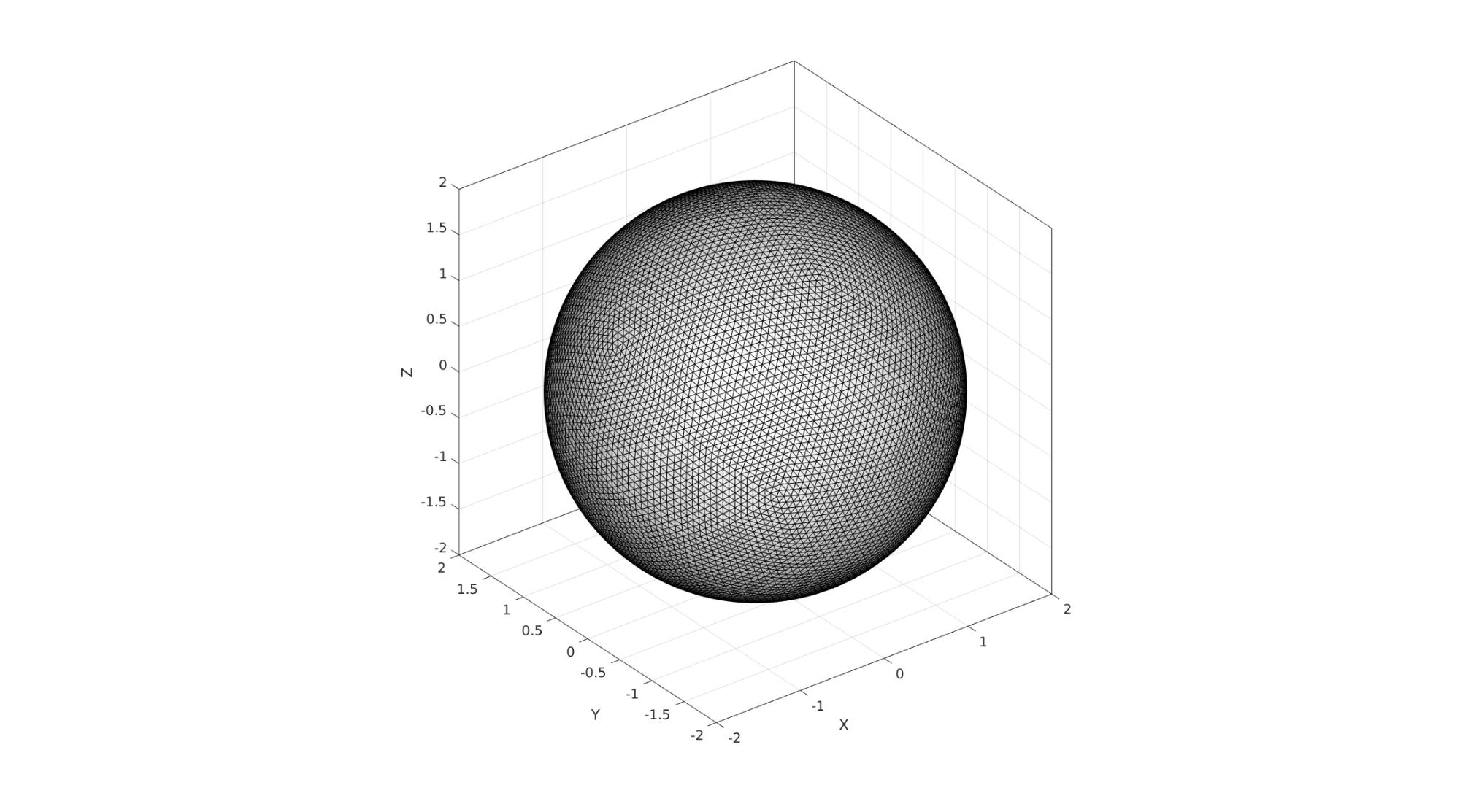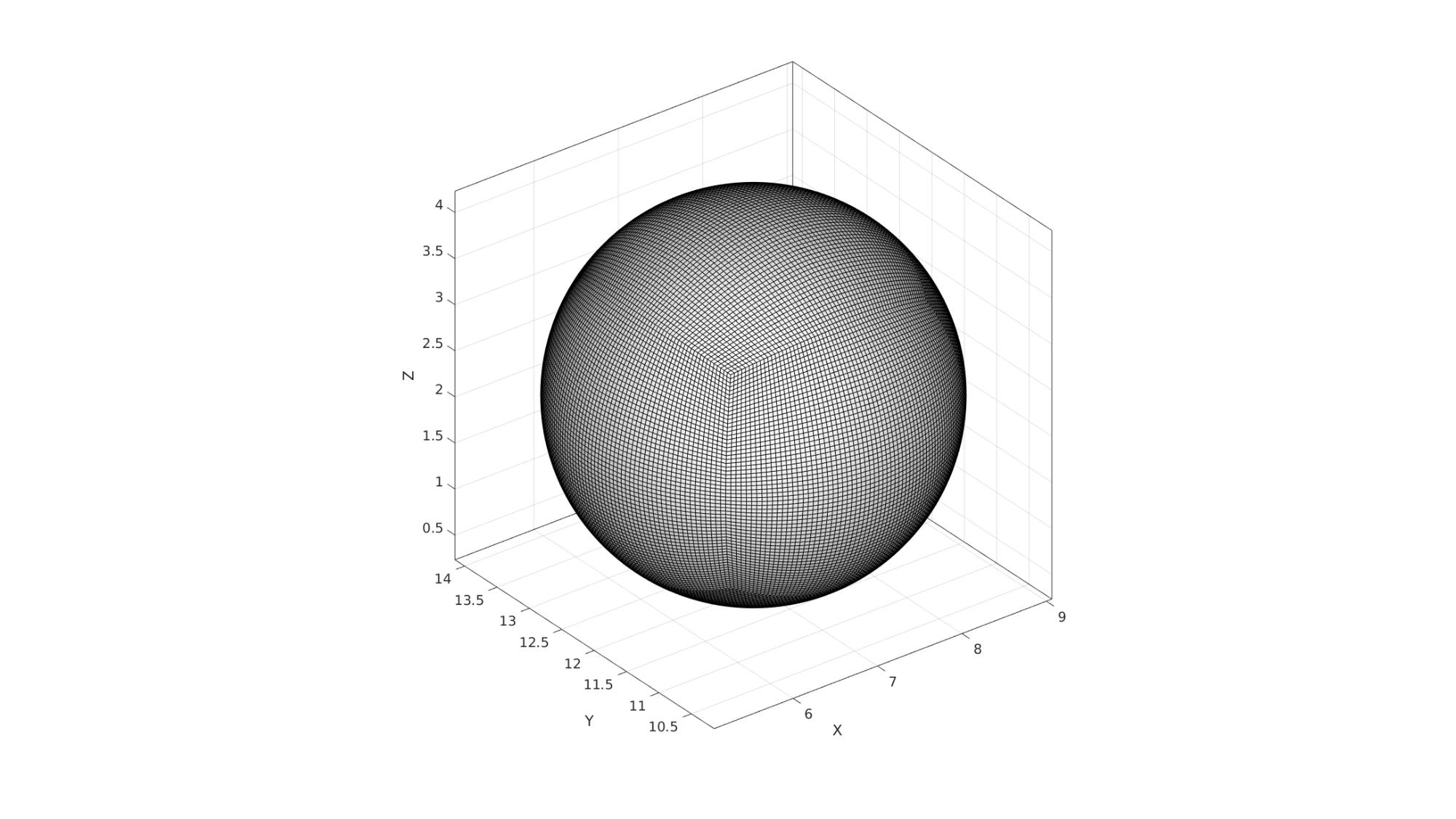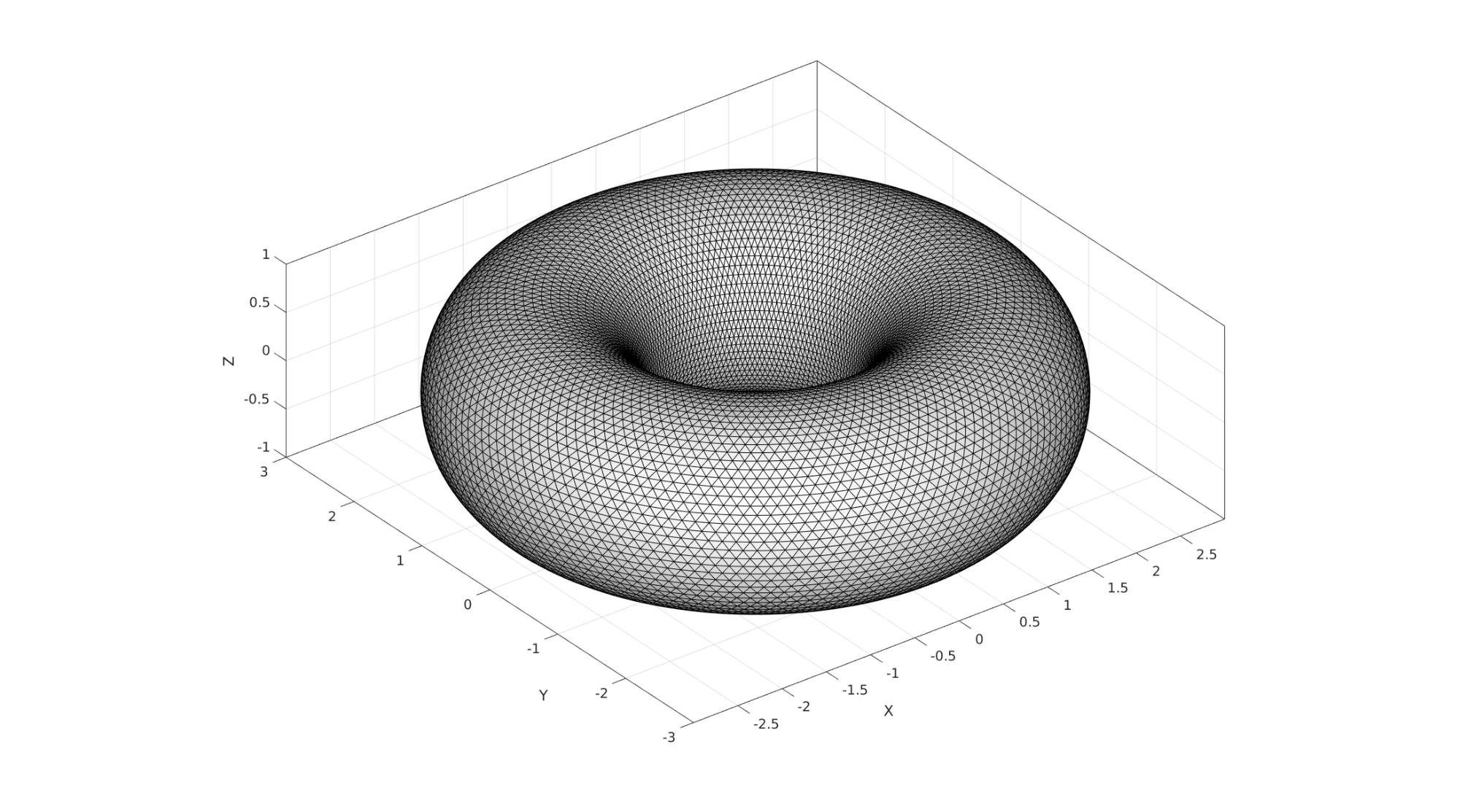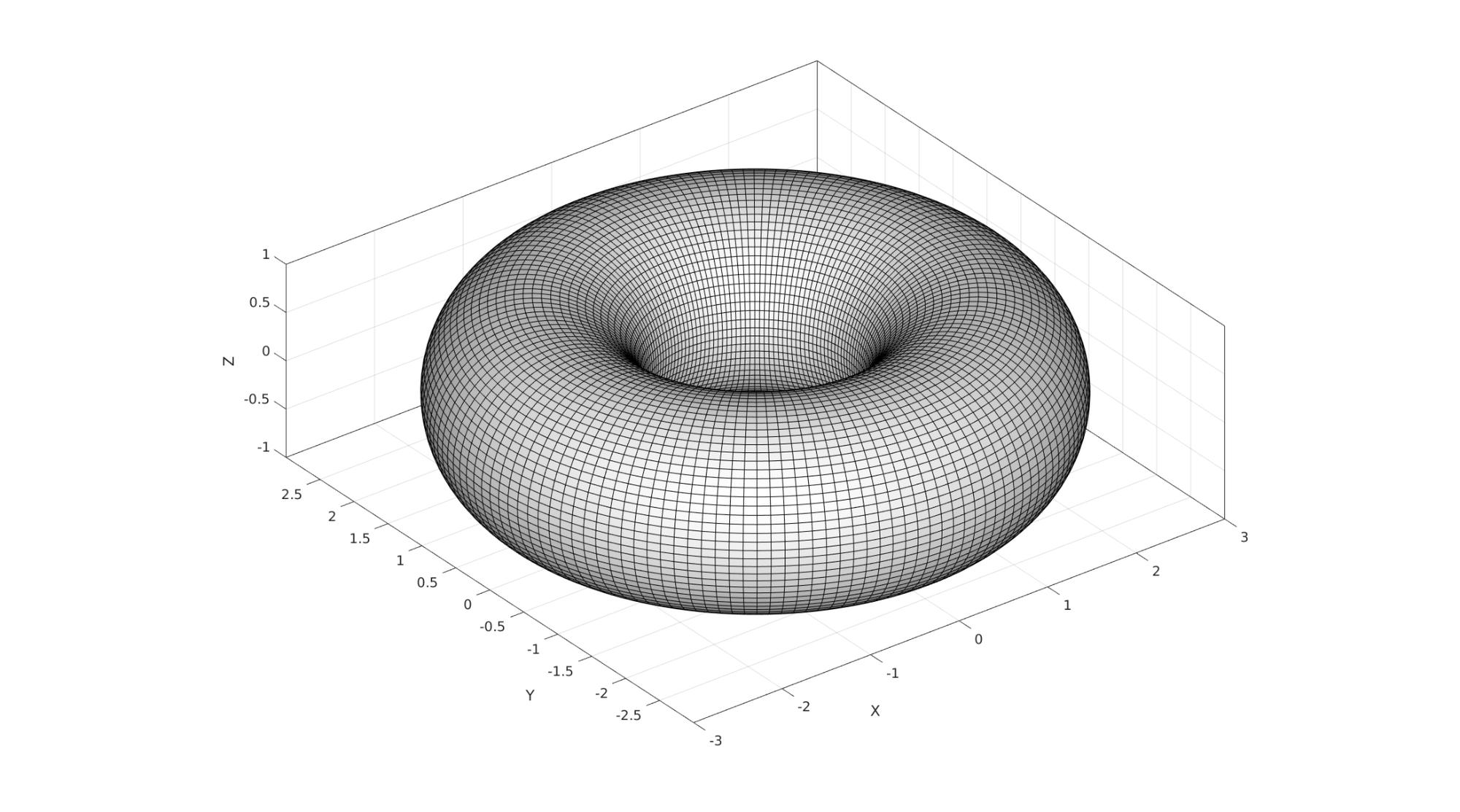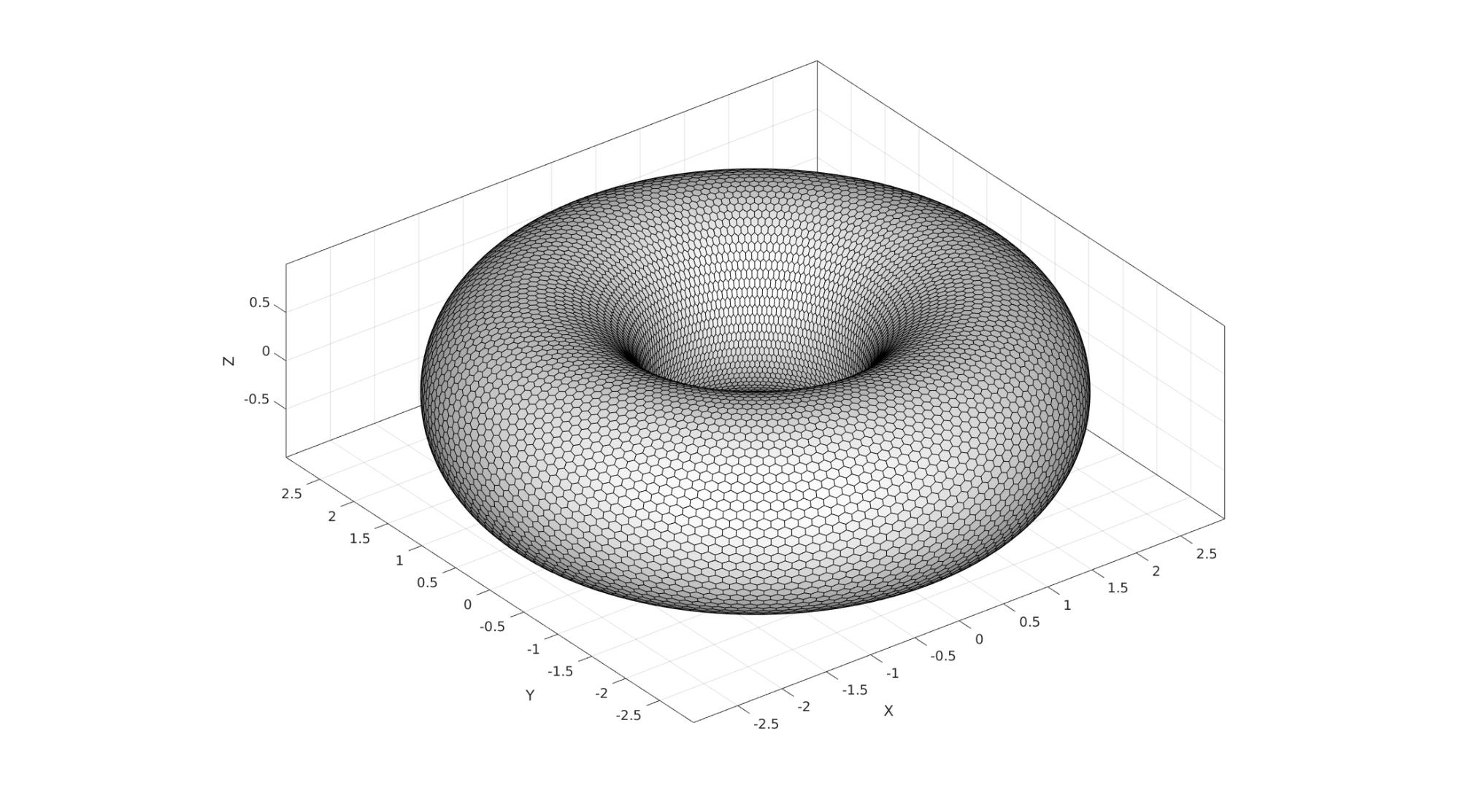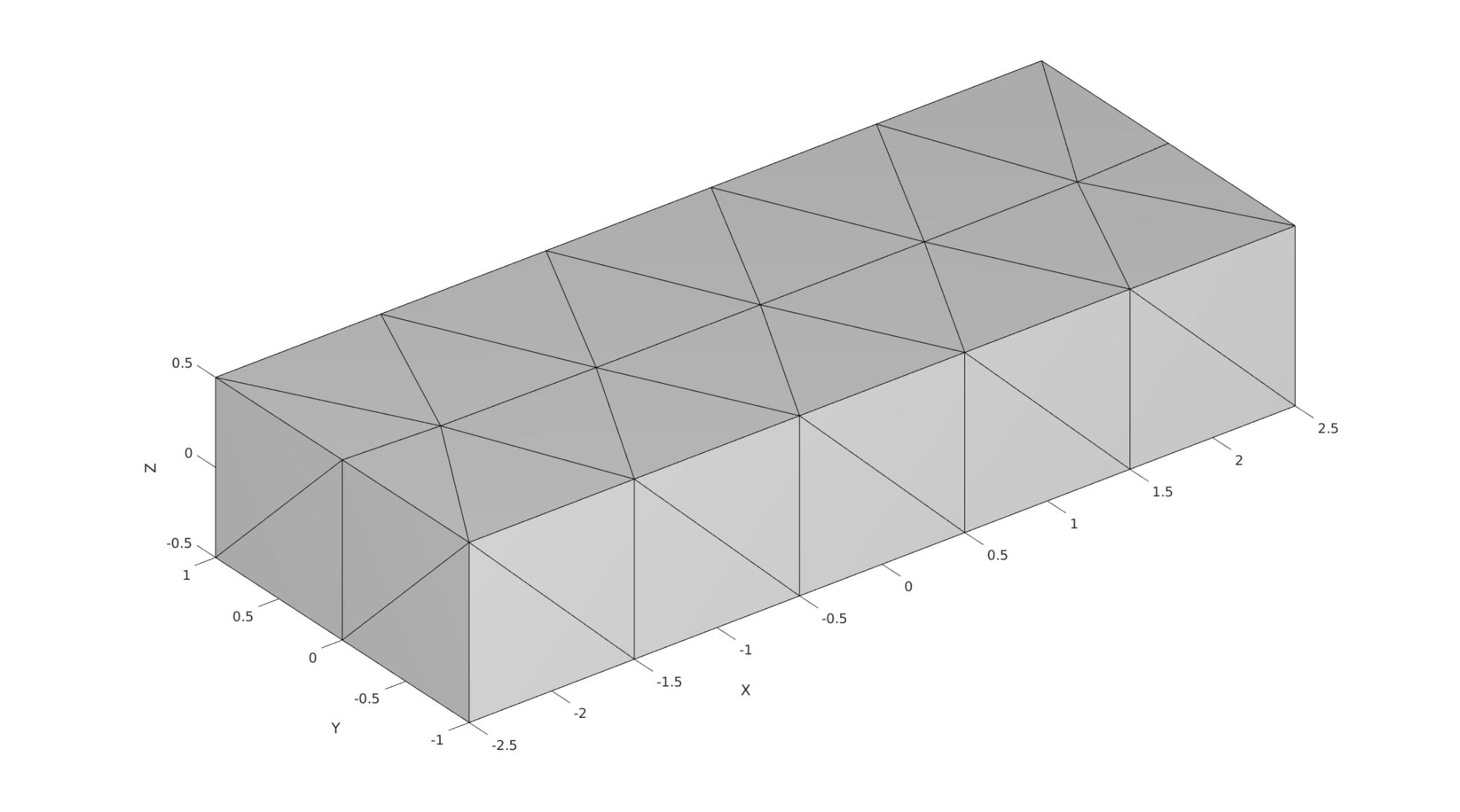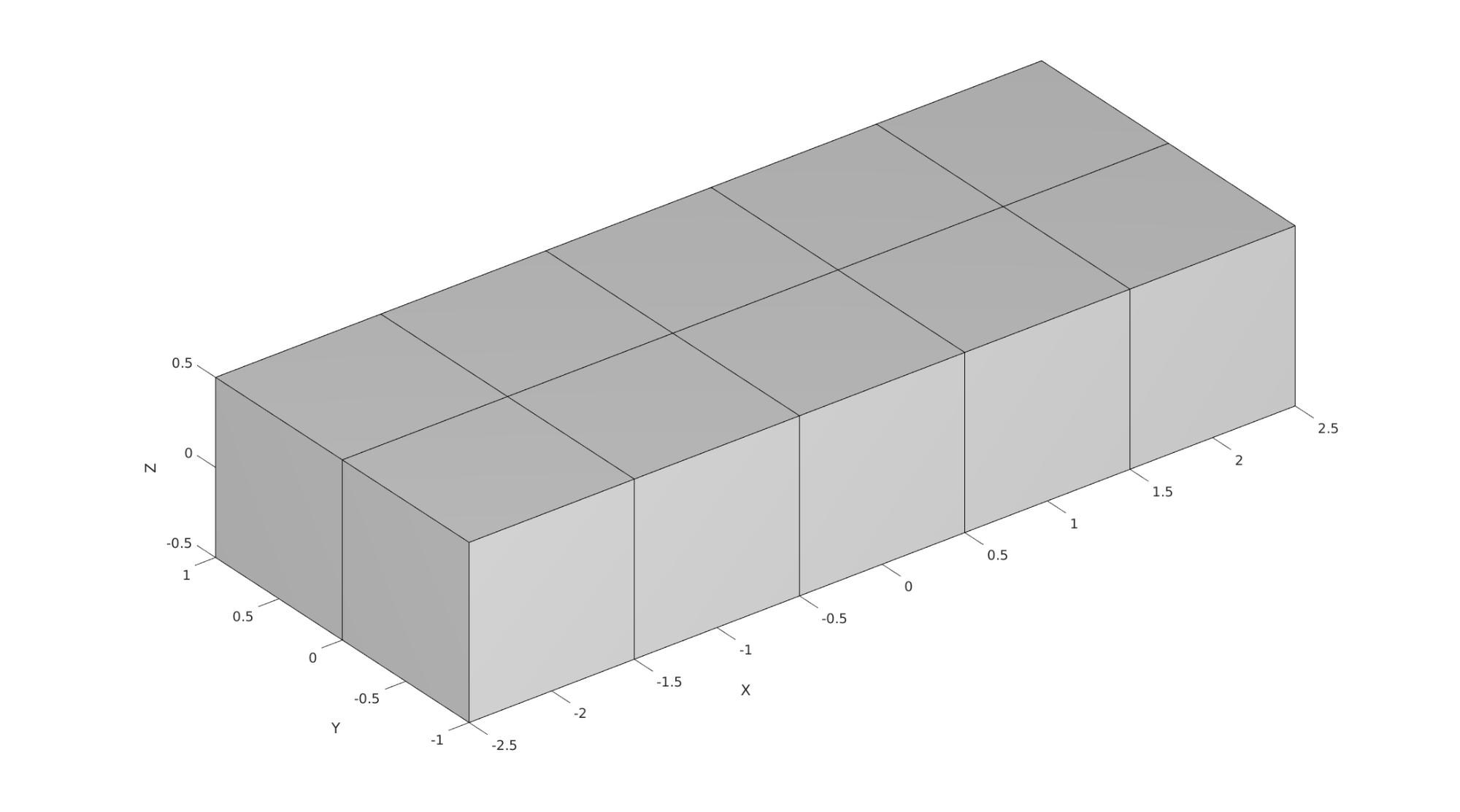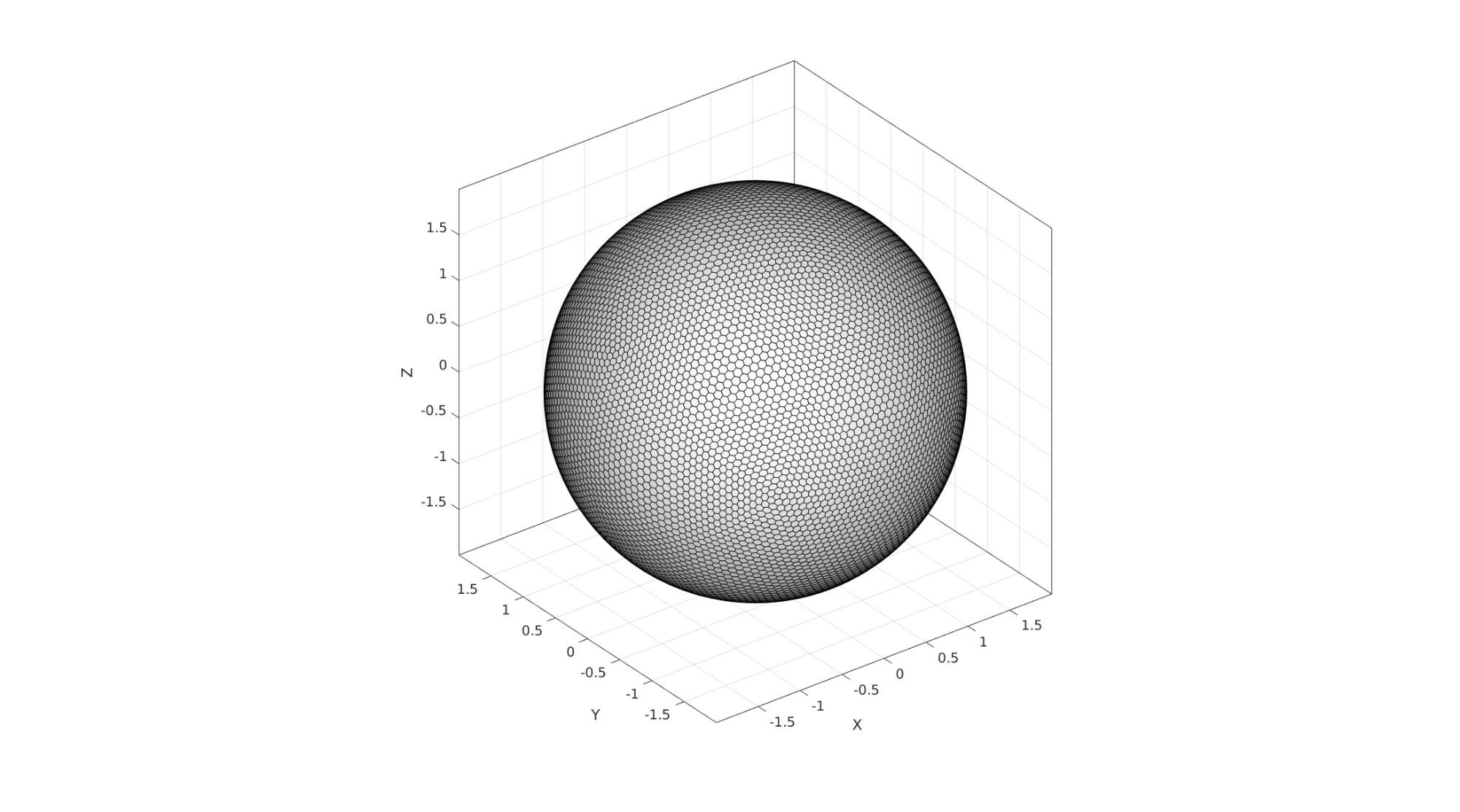```end
```GIBBON www.gibboncode.org

Kevin Mattheus Moerman, [email protected]

GIBBON footer text

GIBBON: The Geometry and Image-based Bioengineering add-On. A toolbox for image segmentation, image-based modeling, meshing, and finite element analysis.

Copyright (C) 2006-2021 Kevin Mattheus Moerman and the GIBBON contributors

This program is free software: you can redistribute it and/or modify it under the terms of the GNU General Public License as published by the Free Software Foundation, either version 3 of the License, or (at your option) any later version.

This program is distributed in the hope that it will be useful, but WITHOUT ANY WARRANTY; without even the implied warranty of MERCHANTABILITY or FITNESS FOR A PARTICULAR PURPOSE. See the GNU General Public License for more details.

You should have received a copy of the GNU General Public License along with this program. If not, see http://www.gnu.org/licenses/.

GIBBON footer text

GIBBON: The Geometry and Image-based Bioengineering add-On. A toolbox for image segmentation, image-based modeling, meshing, and finite element analysis.

Copyright (C) 2006-2023 Kevin Mattheus Moerman and the GIBBON contributors

This program is free software: you can redistribute it and/or modify it under the terms of the GNU General Public License as published by the Free Software Foundation, either version 3 of the License, or (at your option) any later version.

This program is distributed in the hope that it will be useful, but WITHOUT ANY WARRANTY; without even the implied warranty of MERCHANTABILITY or FITNESS FOR A PARTICULAR PURPOSE. See the GNU General Public License for more details.

You should have received a copy of the GNU General Public License along with this program. If not, see http://www.gnu.org/licenses/.